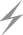Computer Aids for VLSI Design
Steven M. Rubin

Chapter 5: Static Analysis Tools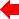Section 5 of 6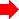## 5.5 Verification

The essential difference between verifiers and rule checkers is that verifiers are given operating requirements to check for each different circuit. Thus any of the previously described rule checkers could be called a verifier if the conditions being checked were explicitly specified as part of the design. However, such conditions do not usually vary between designs because checkers are verifying low-level correctness conditions that vary only with the environment. The verifiers discussed here do the same kind of rule checking, but work with individualized rules for each circuit.

Two types of verifiers will be discussed: timing and functional. Timing verifiers determine the longest delay path in a circuit to optimize performance and to make sure that the clock cycles are correct. Functional verifiers compare symbolic descriptions of circuit functionality with the derived behavior of the individual parts of the circuit, also described symbolically. In each case a specification is given (time delays or behavior) and the checker ensures that the rules are met.

### 5.5.1 Timing Verification

As circuits become complex, it is increasingly difficult to analyze them accurately and completely for precise timing characteristics. Rather than run a circuit simulator such as SPICE [Nagel] that may take weeks to finish, a timing verifier can approximate delays quickly and report much of the same information [Harrison and Olson]. Timing verification is more like electrical-rule checking because its essential function is to traverse the circuit network. For every input and output signal, there are many possible paths through the circuit. Each path consists of a set of network nodes that connect the output of one component to the input of another. If the delay of each node is first determined and stored, then the verifier's job consists of recursively finding the worst-case path to every output:

```   OverallDelay = max(WorstDelay(EachOutputNode))

WorstDelay(Node) =
if Node is INPUT then WorstDelay = 0 else
WorstDelay = ComponentDelay(InputComponent(Node)) +
WireDelay(Node) + max(WorstDelay(EachInputNode(InputComponent(Node))))
```
The delay of nodes and components can be determined in a number of ways. Printed-circuit board components have documented delays that must be combined with wire delays and signal conditions to obtain overall timing ranges [McWilliams]. Integrated-circuit components vary widely in delay and are even data-dependent in some environments such as nMOS. An RC tree, which models the delay of circuit elements with resistance and capacitance, can quickly approximate most timing [Penfield and Rubenstein; Horowitz; Lin and Mead]. RC trees are described in Chapter 6. To determine a path delay precisely, many timing verifiers extract the details of the longest path and use circuit simulation to evaluate the timing fully [Ousterhout; Jouppi].

There are a number of additional considerations that must be addressed to verify accurately the delays in a circuit. As was mentioned before, nMOS components are data-dependent, and therefore a chain of inverters that feed each other should have their delay times redefined, allowing for the fact that every other one will switch much more quickly. Another problem that arises with MOS transistors is that they can function bidirectionally, which means that complex arrays of these components can present an exponential number of paths to the timing verifier. The Crystal system uses user-specified directionality information to reduce the possible paths through a circuit [Ousterhout]. Crystal also has special code to detect buses so that massively connected precharging schemes do not appear to have infinite paths onto and off of the bus. The TV system has complex interconnect rules to determine signal flow directions automatically [Jouppi].

Knowledge of fixed signals can help to limit the search possibilities. For example, clock lines create firewalls throughout the network by removing clocked components from the analysis, since it is known when these components will operate. A difficult problem is the detection of delays that continue to propagate on one clock phase while another is active. These cross-phase signals are common, and should be allowed to continue propagating during the other clock cycle provided that they quiesce before they are used.

One major problem with timing analyzers is their user interface. Since the operation focuses on some subset of the circuit, much help is needed to make it focus correctly. Although intelligent analysis techniques help some, only the user knows what is correct. Thus it is necessary to allow user-specified information such as wire direction, initial conditions, and elimination of circuitry from analysis. The timing analyzer should also be able to indicate paths graphically and to explain why they are critical.

In addition to finding critical-path delays, timing verifiers can be used to do miscellaneous static analysis. For example, there are timing-verification systems that find high-speed components off the critical path that can be slowed down to save power [Jouppi; Trimberger]. One system couples timing analysis with transistor sizing so that it can optimize either time or space [Fishburn and Dunlop]. Once again it is seen that much static analysis can conveniently be done at one time, because the methods overlap. This is also good because the more an analysis tool looks for, the more it knows and is able to analyze.

### 5.5.2 Functional Verification

The last static analysis technique to be discussed is functional verification. Each primitive component can be described behaviorally in terms of its inputs, outputs, and internal state. By combining these component descriptions, an overall circuit behavior is produced that symbolically represents the circuit's function. Verification consists of comparing this derived behavior with a designer-specified behavior. Although not identical, the two descriptions should be mathematically equivalent, in which case the circuit is successfully verified. The three difficult aspects of this process are the selection of a behavioral representation, the aggregation of individual behavioral descriptions to form overall circuit specifications, and the comparison of derived functionality with specified functionality. It is also much harder to verify asynchronous circuits than it is to verify those that are synchronous.

One useful model of circuit behavior consists of equations for the internal state and the component's output [Gordon 81]. These equations use the values of component input and any previous state so that a notion of time is available, much like the temporal logic described in Chapter 2. For unclocked and unbuffered components, there is no state, so these equations are simply formulas that give the output in terms of the input. For example, a NAND gate has no state and is described as:

output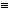input1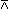input2

Conditional expressions are also available. For example, a multiplexor that chooses between two inputs according to a third selector input is described as:

outputif selector then input1 else input2

Registers that store data must be described with both output equations and state equations. A buffer is:

outputlast memory
memoryinput

Gordon has shown how this scheme can be used in both logic gate and nMOS transistor descriptions. However, the MOS transistor cannot be modeled bidirectionally and so is described as:

drainif gate = 1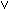last memory = 1 then source else UNDEFINED
memorygate

This retains the state of the output that is held on a wire even after the gate current is cut off.

Combining state and output equations is a simple matter of substitution. The resulting equations are long and complex, but they can be reduced with standard techniques. Special induction methods can be employed at this stage to aggregate multiple parallel bits of processing into multibit values [German and Wang]. When components are connected in a loop, it is the use of clocked registers that helps to break the infinite recursion. This is because clocked components are typically found inside of such loops, which breaks them down to one state and one output specification for the entire loop. It is for this reason that synchronous circuits are easier to verify than are asynchronous ones. Also, during this behavioral-aggregation phase, certain electrical-rule checks can be performed to ensure that the components connect correctly [Barrow].

The final stage of verification compares the aggregated behavior with the user-specified behavior. This involves showing equivalence between two sets of equations, and it can be done in a number of ways [Barrow]. Pure identity is rare but should be checked first. After that, the equations are reduced to canonical form so that they can again be compared. This reduction involves the elimination of constants (A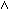1 becomes A) and the rearrangement of clauses into normal conjunctive form ((A(BC)) becomes (AB)(AC)). Another way to test for equivalence is to enumerate all values of variables and compare the results. This works only when the number of inputs and state variables is small.

Besides fully automatic verification techniques, there are methods that involve the user in the proof process. Whereas an automatic verifier finds the equivalence between two sets of equations, a manual verifier checks a user-proposed proof of equivalence [Ketonen and Weening]. In between the two are semiautomatic systems that do what they can, and then refer to the user when they encounter difficulty [Gordon 85].

Although not widely used, functional verification is a significant analysis technique. The mere specification of behavior is a valuable exercise for a designer, and the rigorous analysis of such specifications is very informative. Verification may not become a common design practice, but the use of formal methods is very important and may find acceptance in the design of standard cell libraries, silicon compilers, and other design environments that must combine correctly without constant planning and analysis.Previous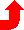Table of Contents NextSteven M. Rubin Static Free Software